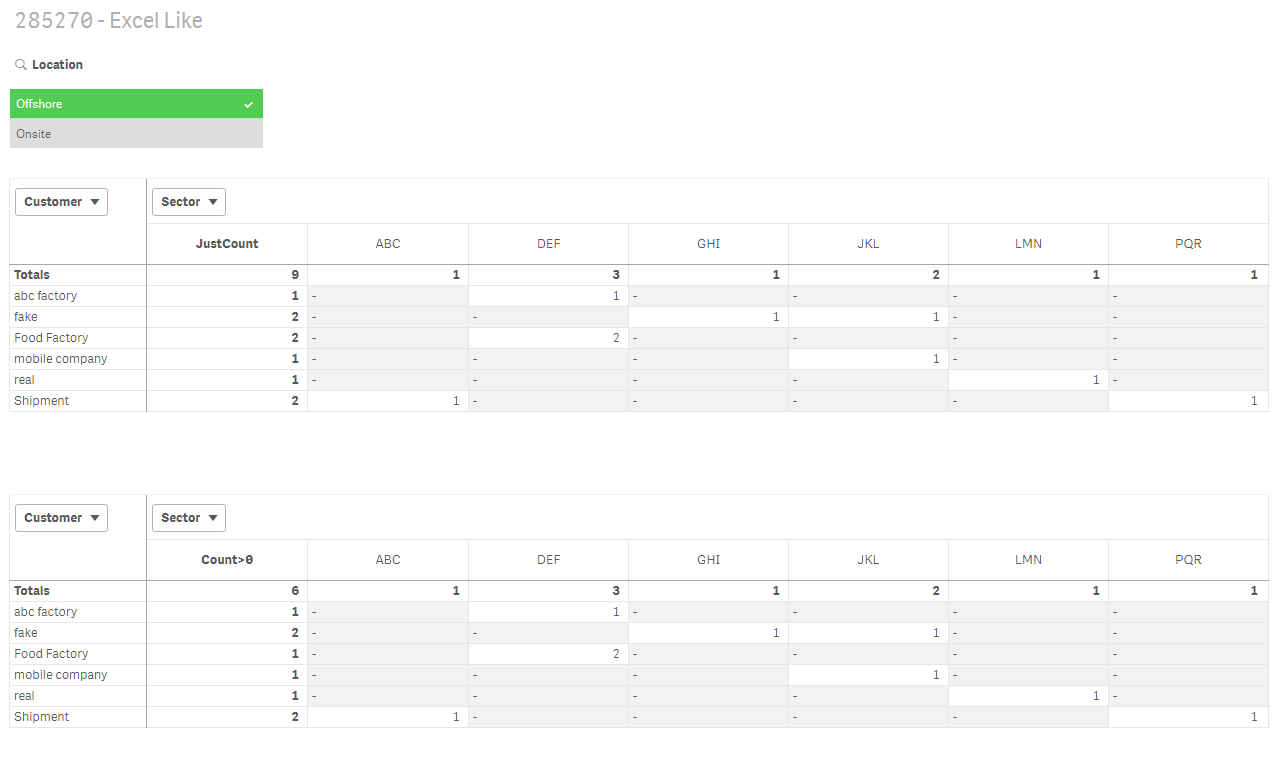# Qlik DataMarket Discussions

Discussion Board for collaboration regarding Qlik DataMarket.

Contributor

## Create expression as per excel in qliksense

Hello,

I have attached a excel in attachments.

My requirement is that i have created a pivot in excel (Sheet 4 tab)I have taken field location as filter.

Customer in rows.

Sector in columns

Count of Empid as values.

Now I have created a new column besides grand total column named as count.

The expression to calculate column COUNT is Countif(range, ">0") where for range i selected from PQR to ABC .

Now after calculating count column i put  filter on it with value greater than 1 as shown belowAfter putting this filter value as greater than 1 i got the total count as 2 (fake and shipment).

I want to implement an expression in qliksense in order to get this count.

Kindly help.

1 Solution

Accepted Solutions
Valued Contributor

## Re: Create expression as per excel in qliksense

=SUM(IF(AGGR(COUNT(DISTINCT Sector),Customer)>1,1,0))15 Replies
Valued Contributor

## Re: Create expression as per excel in qliksense

Hi Pooja,

In order to do that, please do the following:

Create a filter pane or listbox with Location

The create a pivot table with Customer as Row Dimension and Sector as Column Dimension

Then use the following as your measure:

=IF(SecondaryDimensionality()=1,Count([EMP ID]),IF(Dimensionality()=0,COUNT(DISTINCT Sector),AGGR(COUNT(DISTINCT Sector),Customer)))

Then enable Totals for Sector and for Customer,

It should give you this:See that in my count I have 6 and this is because in Excel you're not including Sector ABC, is that on purpose? of was just missed?

I hope this helps

Luis

Contributor

## Re: Create expression as per excel in qliksense

Yeah I was suppose to include ABC as well. I forgot to do that.

But what I need is that I need an expression for calculating that  count in one go...to show it as one parameter in other table.

Valued Contributor

## Re: Create expression as per excel in qliksense

Then you may need this one?

=AGGR(COUNT(DISTINCT Sector),Customer)

Contributor

## Re: Create expression as per excel in qliksense

its not working..i already tried that...

I have created this expression so far

if(sum(if(aggr(Count({\$<[Customer Name]={'abc factory','fake','real'}Sector={'ABC','PQR','DEF'}>}[Emp ID]),[Customer Name],Sector)>0,1,0))>3,1,0)​

Now I want to use this expression inside sum function in order to sum the out put of this expression.

But its showing nested aggr not allowed error.

Valued Contributor

## Re: Create expression as per excel in qliksense

Hi Pooja,

You showed very good explanations in this post. Can you please explain again the final outcome you want? I'm not confused about what you really wantContributor

## Re: Create expression as per excel in qliksense

I wanted to create an expression just like I did in excel to get that count 2 after applying all the logics in excel like taking count(empid) then checking if count(empid)> 0 then 1 else 0 then taking the sum of this count(empid) i.e

sum(if(aggr(count(EMPLID),CUSTOMER_NAME,sector)>0,1,0))

Now then comparing this whole expression if >3 then 1 else 0 i.e.

if(sum(if(aggr(count(EMPLID),CUSTOMER_NAME,Sector)>0,1,0))>3,1,0)

Now I wanted to use this entire expression in sum function in order to sum the individual count of true condition which would come out to be 1 and would give me 2 as total count as I showed in images .

Contributor

## Re: Create expression as per excel in qliksense

How did you create this just count and count> 0 column in pivot.

Valued Contributor

## Re: Create expression as per excel in qliksense

Hi, without the formulas, can you please describe the outcome maybe in a screenshot?

Are you after a KPI? What is the expected result according to the data provided?

Are you after a table? What is the expected result according to the data provided?

I have provided all the numbers you have shown so I really don't understand what you're after.

if it's about a nested Aggr, you already created a post for that.

In this post we're trying to solve the issue you presented

Cheers,

Luis

Valued Contributor

Hi,